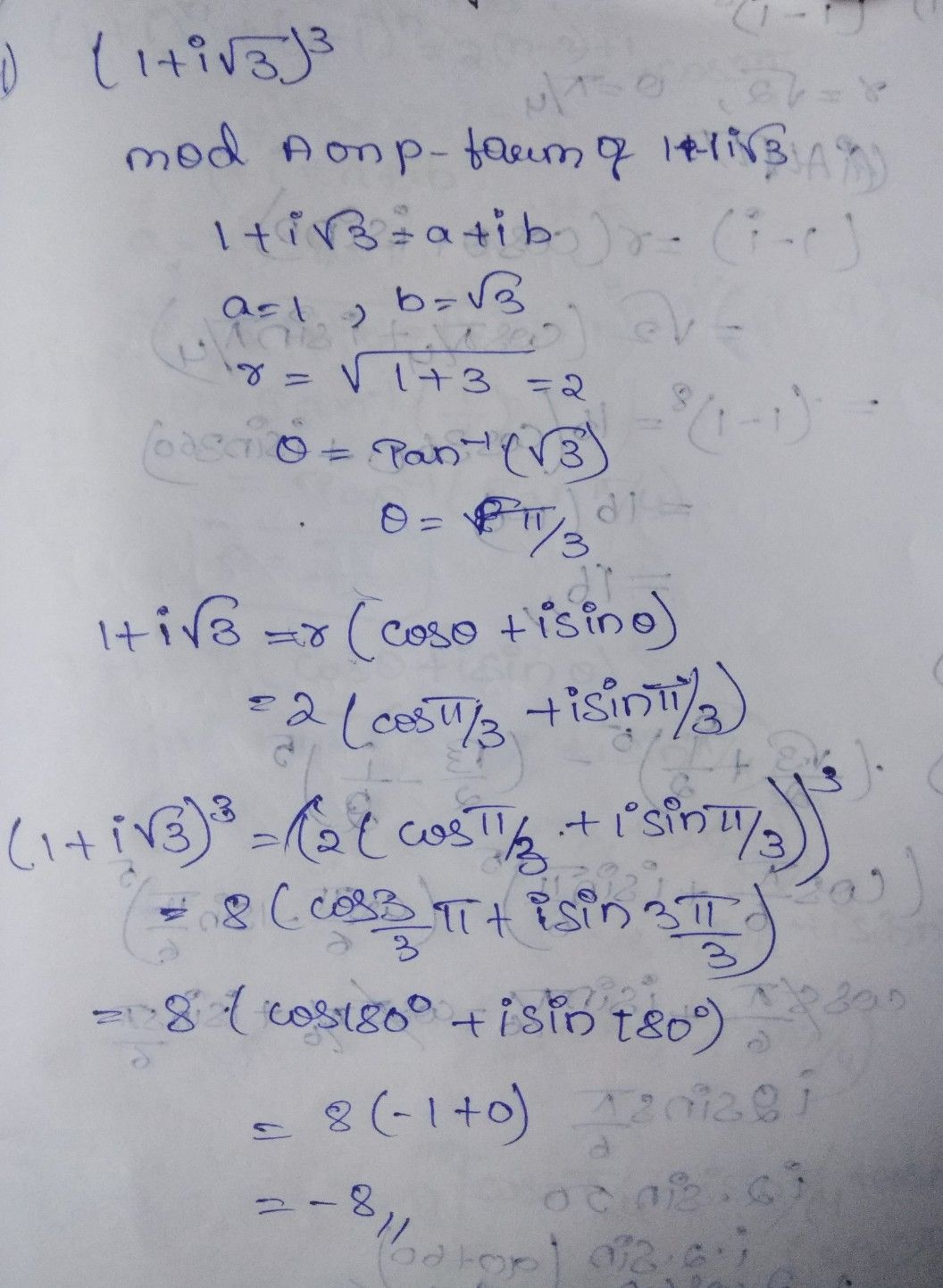Symbol
ProblemFind th volue $0+$ $\left(1+1\sqrt{3\right)} ^{3}$
Calculus
SolutionQanda teacher - A Reddy